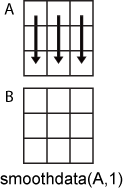smoothdata

说明

B = smoothdata(A) 使用以启发方式确定的固定窗口长度返回向量元素的移动平均值。窗口向下滑动向量的长度，计算每个窗口中的元素的平均值。

• 如果 A 为矩阵，smoothdata 计算每列的移动平均值。

• 如果 A 是多维数组，则 smoothdata 沿大小不等于 1 的第一个维度进行运算。

• 如果 A 是包含数值变量的表或时间表，则 smoothdata 针对每个变量单独执行运算。

B = smoothdata(A,dim) 沿 A 的维度 dim 执行运算。例如，如果 A 是一个矩阵，则 smoothdata(A,2)A 中的每行数据进行平滑处理。

B = smoothdata(___,method) 为上述任一语法指定平滑处理方法。例如，B = smoothdata(A,'sgolay') 使用 Savitzky-golay 滤波器对 A 中的数据进行平滑处理。

B = smoothdata(___,method,window) 指定平滑处理方法使用的窗口长度。例如，smoothdata(A,'movmedian',5) 通过求五元素滑动窗的中位数，来对 A 中的数据进行平滑处理。

B = smoothdata(___,nanflag) 指定在使用上述任一语法时如何处理 NaN 值。'omitnan' 将忽略 NaN 值，'includenan' 则会在每个窗口中进行计算时包含 NaN 值。

B = smoothdata(___,Name,Value) 使用一个或多个名称-值对组参数指定用于平滑处理的其他参数。例如，如果 t 是时间值向量，则 smoothdata(A,'SamplePoints',t) 相对于 t 中的时间对 A 中的数据进行平滑处理。

[B,window] = smoothdata(___) 还会返回移动窗长度。

示例

x = 1:100;
A = cos(2*pi*0.05*x+2*pi*rand) + 0.5*randn(1,100);
B = smoothdata(A);
plot(x,A,'-o',x,B,'-x')
legend('Original Data','Smoothed Data')x = 1:100;
s1 = cos(2*pi*0.03*x+2*pi*rand) + 0.5*randn(1,100);
s2 = cos(2*pi*0.04*x+2*pi*rand) + 0.4*randn(1,100) + 5;
s3 = cos(2*pi*0.05*x+2*pi*rand) + 0.3*randn(1,100) - 5;
A = [s1; s2; s3];
B = smoothdata(A,2);
plot(x,B(1,:),x,B(2,:),x,B(3,:))x = 1:100;
A = cos(2*pi*0.05*x+2*pi*rand) + 0.5*randn(1,100);
[B, window] = smoothdata(A,'gaussian');
window
window = 4

C = smoothdata(A,'gaussian',20);
plot(x,B,'-o',x,C,'-x')
legend('Small Window','Large Window')A = [NaN randn(1,48) NaN randn(1,49) NaN];
B = smoothdata(A);

C = smoothdata(A,'includenan');

plot(1:100,B,'-o',1:100,C,'-x')
legend('Ignore NaN','Include NaN')x = 1:100;
A = cos(2*pi*0.05*x+2*pi*rand) + 0.5*randn(1,100);
t = datetime(2017,1,1,0,0,0) + hours(0:99);
B = smoothdata(A,'SamplePoints',t);
plot(t,A,'-o',t,B,'-x')
legend('Original Data','Smoothed Data')输入参数

B = smoothdata(A,1)A 中的每列数据进行平滑处理。B = smoothdata(A,2)A 中的每行数据进行平滑处理。A 为表或时间表时，不支持 dimsmoothdata 分别对每个表或时间表变量进行运算。

• 'movmean' - A 的每个窗口内的移动平均值。此方法对于减少数据中的周期性趋势很有用。

• 'movmedian' - A 的每个窗口内的移动中位数。当存在离群值时，此方法对于减少数据中的周期性趋势很有用。

• 'gaussian' - A 的每个窗口内的高斯加权移动平均值。

• 'lowess' - A 的每个窗口内的线性回归。该方法可能会耗费大量计算资源，但会减少不连续性。

• 'loess' - A 的每个窗口内的二次回归。此方法的计算开销略高于 'lowess'

• 'rlowess' - A 的每个窗口内的稳健线性回归。此方法比 'lowess' 方法的计算开销更大，不过它在处理离群值时更为稳健。

• 'rloess' - A 的每个窗口内的稳健二次回归。此方法比 'loess' 方法的计算开销更大，不过它在处理离群值时更为稳健。

• 'sgolay' - Savitzky-Golay 滤波器，它根据在 A 的每个窗口上拟合的二次多项式进行平滑处理。当数据变化很快时，此方法可能比其他方法更有效。

A 是时间表或 'SamplePoints' 指定为 datetimeduration 向量时，window 的类型必须为 duration，并相对于样本点计算窗口。

NaN 条件，指定为下列值之一：

• 'omitnan' - 忽略输入中的 NaN 值。如果窗口所包含的值全部为 NaN，则 smoothdata 返回 NaN

• 'includenan' - 在每个窗口内计算时包括 NaN 值，得到 NaN

名称-值对组参数

'Var1'

"Var1"

{'Var1' 'Var2'}

["Var1" "Var2"]

1

[1 3 5]

[true false true]

@isnumeric

vartype 下标

vartype 函数生成的表下标

vartype('numeric')

Savitzky-golay 阶数，指定为包含 'Degree' 和非负整数的逗号分隔对组。仅当指定的平滑处理方法为 'sgolay' 时，才能指定此名称-值对组。'Degree' 的值对应于 Savitzky-golay 滤波器中多项式的次数，该次数适合每个窗口内的数据，默认为 2。

输出参数

window 指定为输入参数时，输出值与输入值匹配。当 window 未指定为输入参数时，其值是由 smoothdata 基于输入数据启发性确定的标量。